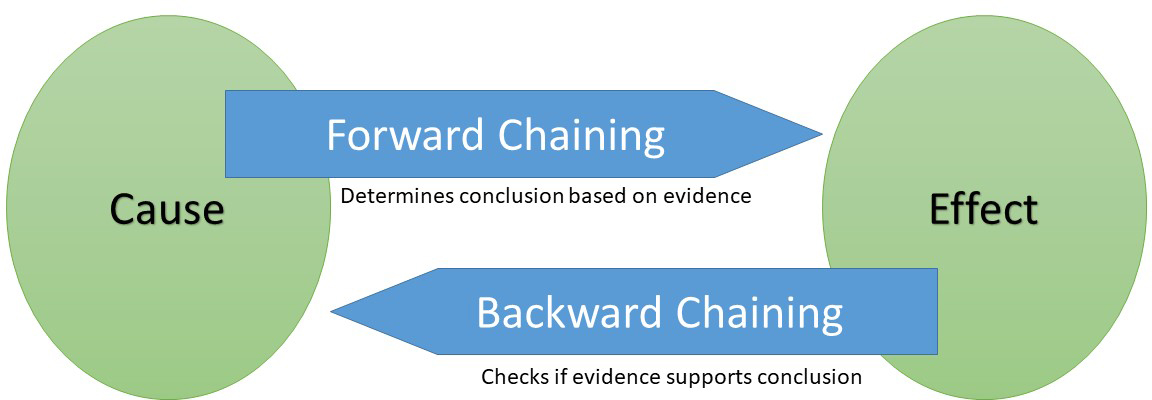# Argonne National Laboratory

Nuclear Science and Engineering

# AI/ML Automated Reasoning

How to have the machine think” like a human

Automated reasoning methods provide a formal means for inferring the status of a system given a set of hypothesized relationships among system variables. Each of these relationships is individually held up for inspection as to its validity given measurements of the variables. The inference regarding system status involves the application of inferential logic to determine what the individual truths imply about the overall system. This inference is performed by a reasoning engine, which is its own discipline in the field of computer science.

These hypothesized relationships when based on physics are derived from the laws of conservation of mass, energy and momentum. The relationships can be either of a qualitative or quantitative nature. Each type is illustrated below.

### Qualitative Reasoning

The method of qualitative automated reasoning can be used to evaluate a set of conditional logic statements written for a system to infer what collectively the statements imply for the system. Logic statements written for a component in the system appear in a form where the antecedent is the trend of a process variable in time and the consequent is the component fault status. The qualitative aspect arises from antecedents that are written as process variables of a type having values increasing, decreasing, or no change. The relationship between the trend and fault status is given by the conservation balances for the component that are represented by a qualitative model. A forward chaining algorithm is used to make the inference.The method of qualitative automated reasoning operates on conditional logic statements that represent the status of a component. A conservation-based model associates process variable trends with the fault status of the component. The qualitative aspect of the model derives from the fact that variables are not continuous but assume discrete values.

As an example of such a model, consider a valve in a pipe leg of a thermal hydraulic system. A full set of instrumentation on the valve would measure outlet and inlet pressures Po and Pi, respectively, and flow w, and valve stem position. Typically, there will be some subset of instrumentation on this component. An example of a conditional statement for this component based on the conservation of momentum is

(Pi - Po)↑  w↓  ⇒  Qnom↑

which is a test for a momentum imbalance that if true is indicative of a reduction in hydraulic resistance. A companion conditional statement is

(Pi - Po)↑ w↓  ⇒  Qnom↓

which if true is indicative of an increase in hydraulic resistance. In the above the variable Qnom can assume a value of increasing”, decreasing”, or constant”. It represents the status of fluid momentum in the control volume enclosing the valve compared to the normal un-faulted operating condition.

### Quantitative Reasoning

The method of quantitative automated reasoning can be used to evaluate a set of conditional logic statements written for a system to infer what the statements collectively imply for the system. The method parallels that of qualitative automated reasoning except that the antecedent is real valued function formed from a first principles analytic estimate obtained from a quantitative model.

In the quantitative reasoning approach, logic statements describing the quantitative behavior of the process variables of a component are written based on the conservation laws. This includes additional physics compared to the qualitative approach. The writing of these equations is illustrated for the case of a valve where the conservation of momentum yields

Pi - Po = K(w, x) wn

where K is the loss coefficient whose value depends on flowrate and valve stem position x and n depends on the flow regime.

A logic statement for use in quantitative reasoning can be formulated using an overdetermined set of conservation equations and measurements.  The idea is to create a form of analytic redundancy that can detect and identify process parameters and process variables whose calculated or measured values are inconsistent (and in error) compared to their physical values.  Then the actual values can be estimated using a process of logical deduction applied to this overdetermined information set.